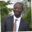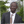Trusted answers to developer questions
Trusted Answers to Developer Questions

Related Tags

algorithm
javascript
programming
communitycreator

# What is the FizzBuzz algorithm in JavaScript?Abel Lifaefi Mbula

Grokking Modern System Design Interview for Engineers & Managers

Ace your System Design Interview and take your career to the next level. Learn to handle the design of applications like Netflix, Quora, Facebook, Uber, and many more in a 45-min interview. Learn the RESHADED framework for architecting web-scale applications by determining requirements, constraints, and assumptions before diving into a step-by-step design process.

### Overview

Writing algorithms is one of the fundamental skills every software developer needs to know to write interesting and important programs.

To recall the definition, we can say that an algorithm is a set of steps for a computer program to accomplish a task.

The more we practice algorithm and data structure challenges, the more we’ll be better at our favorite language, and the more we’ll be able to build solid software programs.

In this shot, we’ll learn to solve a simple but popular programming challenge called FizzBuzz in JavaScript.

### Problem statement

Given an integer n, return a string array, answer (1-indexed) where:

• answer[i] == "FizzBuzz" if i is divisible by 3 and 5.
• answer[i] == "Fizz" if i is divisible by 3.
• answer[i] == "Buzz" if i is divisible by 5.
• answer[i] == i (as a string) if none of the above conditions are true.

### Examples

Input: n = 3

Output: [“1”,“2”,“Fizz”]

Input: n = 5

Output: [“1”,“2”,“Fizz”,“4”,“Buzz”]

Input: n = 15

Output: [“1”,“2”,“Fizz”,“4”,“Buzz”,“Fizz”,“7”,“8”,“Fizz”,“Buzz”,“11”,“Fizz”,“13”,“14”,“FizzBuzz”]

### Pseudocode

Let’s start with a pseudocode implementation of this problem:

Set result[]
for numbers from 1 to n
if number is divisible by 3 and by 5
add "FizzBuzz" to result[]
else if number is divisible by 3
add "Fizz" to result[]
else if number is divisible by 5
add "Buzzz" to result[]
else
add number to result[]
endif
endfor
print result[]


### Flowchart

Let’s look at the flowchart below:

FizzBuzz flowchart

The flowchart represents a very basic implementation of FizzBuzz where the user submits a number and the program print the expected result.

Now that we understand the algorithm, let’s implement it in JavaScript.

### Implementation

Let’s put it all together and run the complete code:

/** * @param {number} n * @return {string[]} * @author Abel L Mbula */function fizzBuzz (n) {    const answer = []        for (let i = 1; i <= n; i++) {        if (i % 3 == 0 && i % 5 == 0)            answer.push("FizzBuzz")        else if (i % 3 == 0)            answer.push("Fizz")        else if (i % 5 == 0)            answer.push("Buzz")        else            answer.push(i.toString())    }        return answer};console.log(fizzBuzz(14))
FizzBuzz in JavaScript

### Explanation

• Line 1 - Line 2: We create the function, fizzbuzz, and an empty array answer, which is returned as output.

• Line 9: We create a for loop that goes from 1 to n.

• Line 10 - Line 17: Inside the loop, we create conditional statements with if-else. In each if statement, we send the result to the array we created at the beginning (answer.push()). Because we need an array of strings, we use .toString() to convert a number to a string.

Note: Most people fail this algorithm challenge because they are tempted to start by checking individual numbers first. Such a solution will work well for numbers less than 15. To have a bug-free solution, we need to put the most specific conditions on the top and the most generic ones on the bottom.

RELATED TAGS

algorithm
javascript
programming
communitycreator

CONTRIBUTORAbel Lifaefi Mbula

Grokking Modern System Design Interview for Engineers & Managers

Ace your System Design Interview and take your career to the next level. Learn to handle the design of applications like Netflix, Quora, Facebook, Uber, and many more in a 45-min interview. Learn the RESHADED framework for architecting web-scale applications by determining requirements, constraints, and assumptions before diving into a step-by-step design process.

Keep Exploring

Learn in-demand tech skills in half the time